# RTD and thermocouple circuits with millivolt calculations

## Instrumentation and Process Control

• #### Question 1

Calculate the amount of voltage seen’’ by the voltmeter given the following measurement and reference junction temperatures: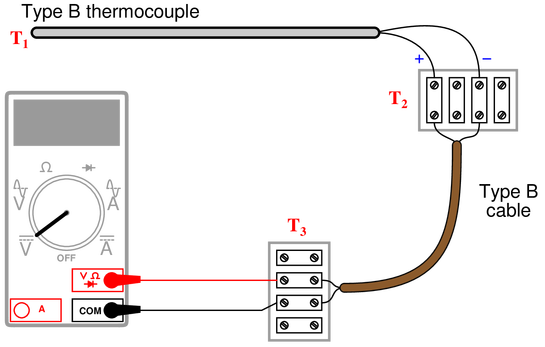{\bullet} $T_{1}$ = 589 $^{o}$F ; $T_{2}$ = 63 $^{o}$F ; $T_3$ = 70 $^{o}$F ; $V_{meter}$ =

{\bullet} $T_{1}$ = 821 $^{o}$F ; $T_{2}$ = 69 $^{o}$F ; $T_3$ = 73 $^{o}$F ; $V_{meter}$ =

{\bullet} $T_{1}$ = 1524 $^{o}$F ; $T_{2}$ = 91 $^{o}$F ; $T_3$ = 105 $^{o}$F ; $V_{meter}$ =

{\bullet} $T_{1}$ = 1922 $^{o}$F ; $T_{2}$ = 102 $^{o}$F ; $T_3$ = 135 $^{o}$F ; $V_{meter}$ =

• #### Question 2

This thermocouple is installed improperly: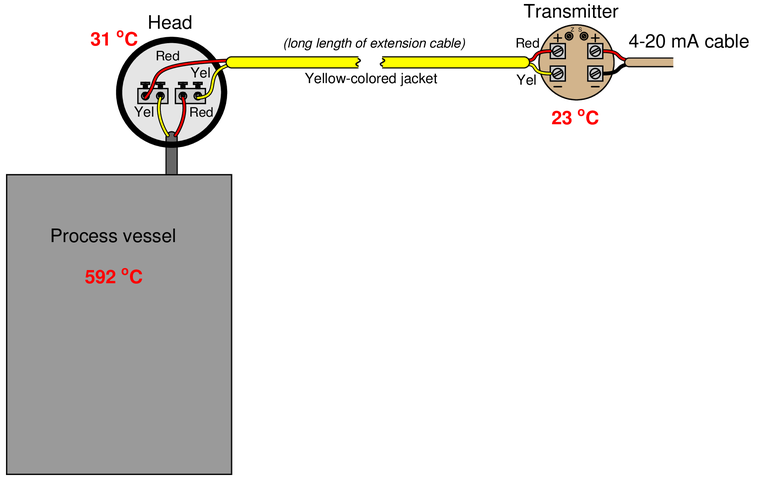Identify the nature of the problem, and then calculate the millivoltage seen at the screw terminals of the temperature transmitter given the temperatures shown. After that, calculate the temperature interpreted by the transmitter assuming it has cold junction compensation (CJC) enabled.

• #### Question 3

Part of this solar-heating control system uses a {\it dual-thermocouple} circuit to compare the temperature inside the solar collector against the temperature inside the house, preventing the circulation fan from running if the house is ever warmer than the collector: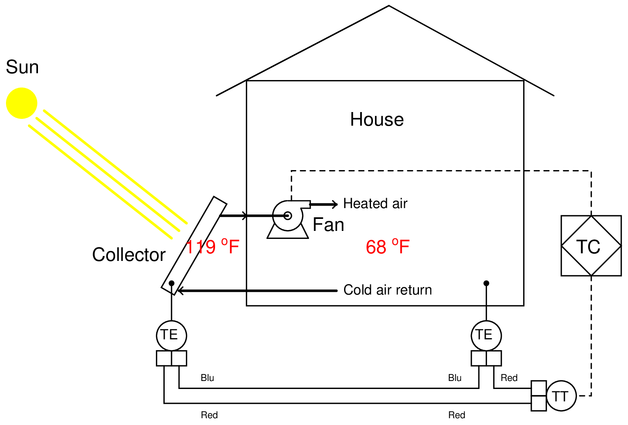{\bullet} Identify the thermocouple type used in this application, and determine whether or not this type is a good choice.

{\bullet} Calculate the voltage measured at the input terminals of the transmitter, and also determine whether or not this transmitter needs to be enabled for reference junction compensation.

{\bullet} Identify the meaning of the diamond-shaped PID symbol labeled TC’‘.

• #### Question 4

Calculate the voltage sensed by the analog-to-digital converter inside the temperature transmitter: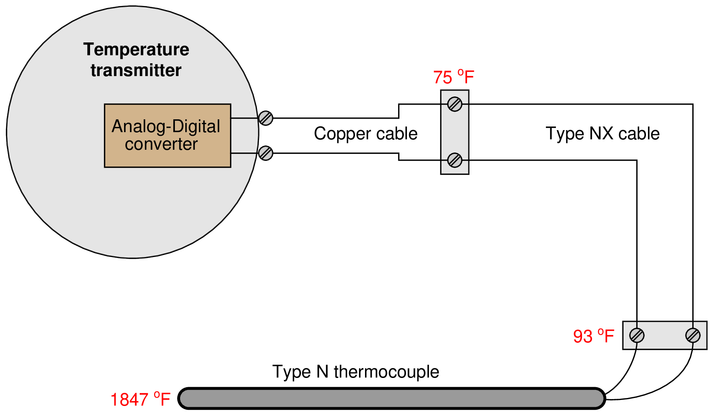• #### Question 5

Calculate the appropriate millivoltage values and potentiometer resistances to simulate a thermocouple at the desired temperatures, assuming the ambient temperature at the transmitter is 72 $^{o}$F and the transmitter has cold junction compensation {\it enabled}: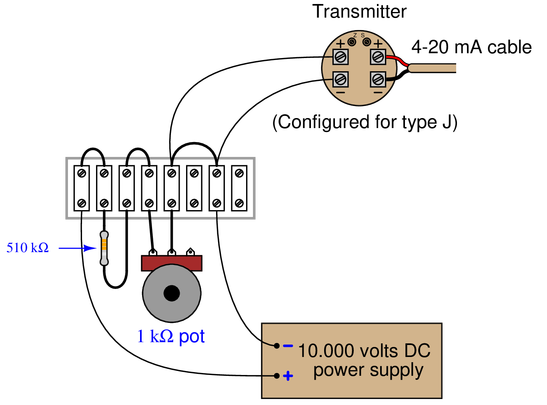{\bullet} $T_{simulate}$ = 550 $^{o}$F ; $V_{input}$ =

{\bullet} $T_{simulate}$ = 300 $^{o}$F ; $V_{input}$ =

{\bullet} $T_{simulate}$ = 150 $^{o}$F ; $V_{input}$ =

• #### Question 6

Suppose you walk up to this thermocouple, installed to measure the temperature of an enclosed process vessel, and connect a sensitive voltmeter to the terminals at the junction head: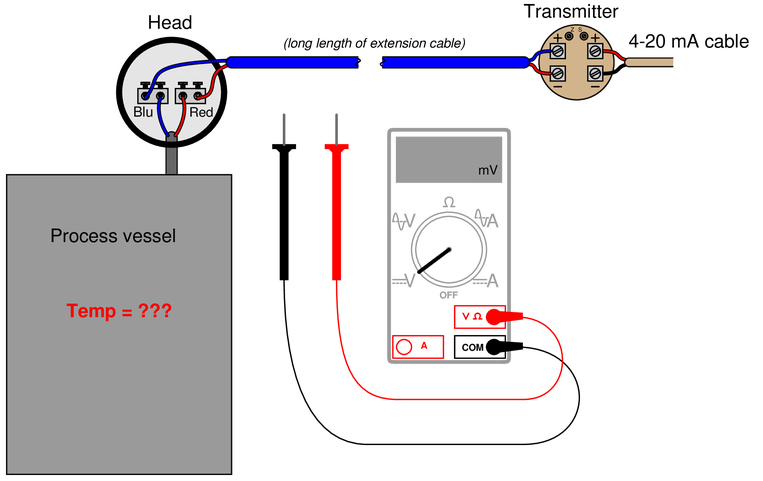Determine the process temperature if you read 7.825 millivolts with the voltmeter connected to the screw terminals inside the thermocouple head. Assume a head temperature of 92 $^{o}$ F.

Suppose at some later time you connected the voltmeter to the transmitter’s input terminals and read 8.332 millivolts. Calculate the process temperature at this time, assuming an ambient temperature of 66 $^{o}$F at the transmitter.

• #### Question 7

A type J thermocouple is inserted into a process, with a digital multimeter connected to its terminals. The ambient temperature at the DMM’s test lead connections is 17 $^{o}$C. Calculate the thermocouple’s measurement junction temperature at the following millivolt measurements (rounding to the nearest degree Celsius):

{\bullet} 5.05 mV ; $T$ =

{\bullet} 17.82 mV ; $T$ =

{\bullet} 31.44 mV ; $T$ =

{\bullet} 40.29 mV ; $T$ =

• #### Question 8

A type K thermocouple is inserted into a process, with a digital multimeter connected to its terminals. The ambient temperature at the DMM’s test lead connections is 84 $^{o}$F. Calculate the thermocouple’s measurement junction temperature at the following millivolt measurements (rounding to the nearest degree Fahrenheit):

{\bullet} 2.55 mV ; $T$ =

{\bullet} 6.21 mV ; $T$ =

{\bullet} 10.93 mV ; $T$ =

{\bullet} 18.83 mV ; $T$ =

• #### Question 9

Suppose three different voltmeters are connected to a thermocouple, with each voltmeter having a different ambient temperature:Calculate the amount of voltage registered by each of the three voltmeters, as well as answer the following questions:

{\bullet} If a fourth voltmeter were connected to this same thermocouple, would it affect the readings of the other three?

{\bullet} If a thermocouple transmitter were connected to this thermocouple, would it affect the readings of the voltmeters? Would it matter whether or not this transmitter were equipped with reference junction compensation?

• #### Question 10

Calculate the amount of voltage seen’’ by the voltmeter given the following measurement and reference junction temperatures: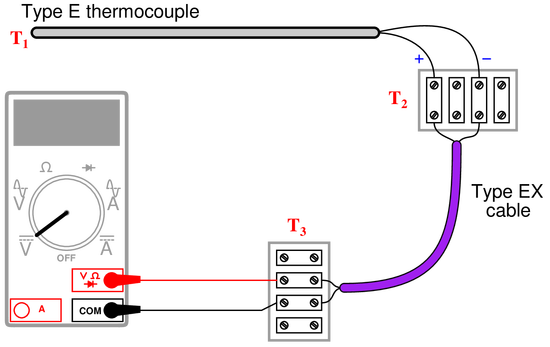{\bullet} $T_{1}$ = 233 $^{o}$C ; $T_{2}$ = 31 $^{o}$C ; $T_3$ = 25 $^{o}$C ; $V_{meter}$ =

{\bullet} $T_{1}$ = 348 $^{o}$C ; $T_{2}$ = 40 $^{o}$C ; $T_3$ = 16 $^{o}$C ; $V_{meter}$ =

{\bullet} $T_{1}$ = $-161$ $^{o}$C ; $T_{2}$ = $-4$ $^{o}$C ; $T_3$ = 23 $^{o}$C ; $V_{meter}$ =

{\bullet} $T_{1}$ = 836 $^{o}$C ; $T_{2}$ = 34 $^{o}$C ; $T_3$ = 19 $^{o}$C ; $V_{meter}$ =

• #### Question 11

Calculate the voltage across the bridge ($V_{AB}$) at the following RTD temperatures, assuming a 604 $\Omega$ nickel-iron RTD with an alpha value of 0.00518:{\bullet} $T$ = 0 $^{o}$C ; $V_{AB}$ =

{\bullet} $T$ = 81 $^{o}$C ; $V_{AB}$ =

{\bullet} $T$ = -45 $^{o}$C ; $V_{AB}$ =

{\bullet} $T$ = 320 $^{o}$F ; $V_{AB}$ =

• #### Question 12

Suppose someone builds a dual-junction thermocouple circuit using type T thermocouple wire (copper and constantan metals), then measures voltage in the loop using a voltmeter: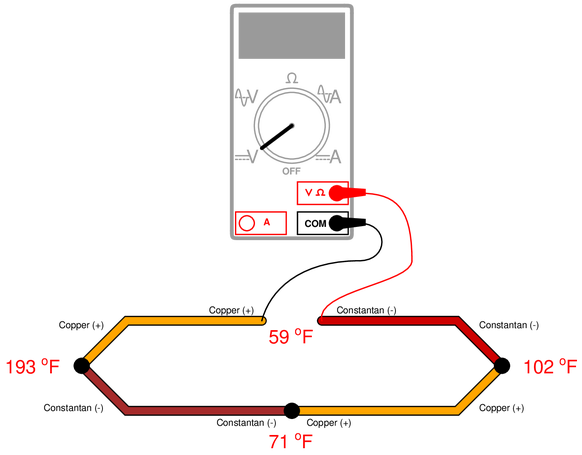Calculate the voltage read by the voltmeter, using a type T thermocouple table to find millivolt potentials for each of the junctions.

• #### Question 13

When a type T’’ thermocouple (copper/constantan) is connected to a voltmeter made of copper wires, two active junctions are formed: one at the point of measurement (the measurement junction) and one at a terminal near the voltmeter (the reference junction). The copper-to-copper junction at the top screw of the terminal block is of no consequence because it is a junction of identical metals, and as such generates no thermoelectric voltage: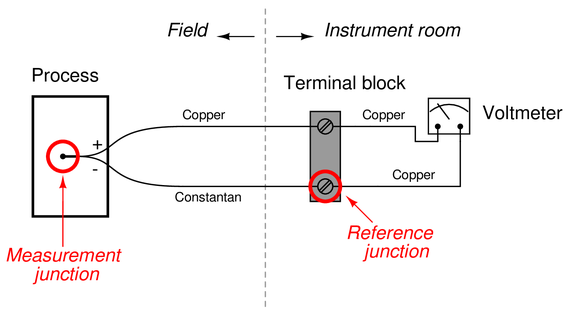The amount of voltage sensed by the voltmeter in this thermocouple circuit is equal to the difference in voltages produced by the measurement and reference junctions:

$$E_{meter} = E_{meas} - E_{ref}$$

Now consider a type J’’ thermocouple connected to a copper-wire voltmeter. Here we see there are not two but {\it three} active junctions of dissimilar metals:Upon first observation it would appear this circuit is more complicated than the type T’’ thermocouple circuit, owing to the existence of the additional dissimilar-metal junction. However, a principle called the {\it Law of Intermediate Metals} allows us to consider the two reference junctions (iron-copper and constantan-copper) as electrically equivalent to a single reference junction of iron-constantan, such that the type J’’ thermocouple circuit becomes just as simple as the type T’’ circuit with one measurement junction and one reference junction in opposition to each other.

Explain what the {\it Law of Intermediate Metals} is, and how it may be used to simplify the two active reference junctions of the type J’’ circuit. Also, explain why it is important that the terminal block be {\it isothermal} in nature.

• #### Question 14

Suppose someone builds a dual-junction thermocouple circuit using type T thermocouple wire (copper and constantan metals), then measures voltage between the two junctions with a voltmeter: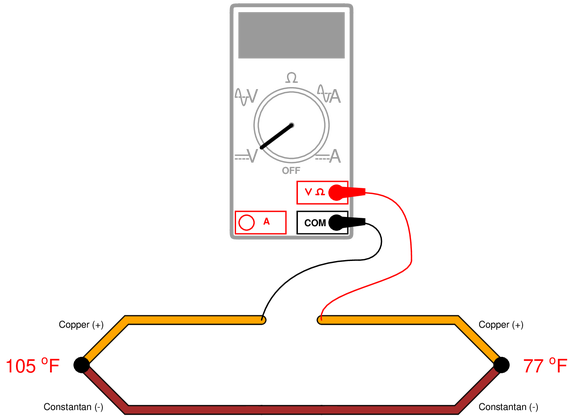Calculate the voltage read by the voltmeter, using a type T thermocouple table to find millivolt potentials for each of the junctions.

• #### Question 15

Determine the temperature of the RTD, given $V_{CD}$ = 140.5 millivolts and $V_{EF}$ = 140.1 millivolts:Assume a 1 k$\Omega$ RTD with $\alpha = 0.00385$, and all wire resistances completely unknown (not assumed to be equal).

• #### Question 16

Suppose you need to measure the temperature of an operating oven using nothing but a 1000 $\Omega$ RTD ($\alpha$ = 0.00385), a 1.75 k$\Omega$ precision resistor, and a battery of unknown voltage: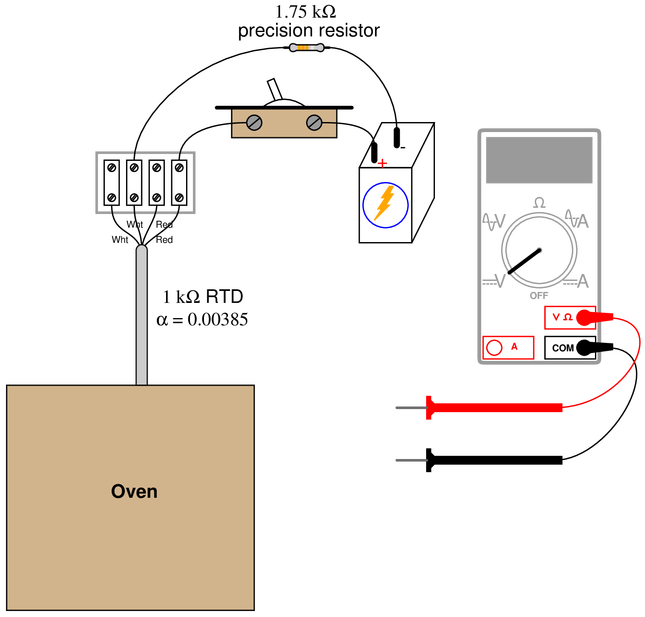Turning the switch on, you measure 1.32 volts across the resistor and 1.22 volts across the RTD. Calculate the oven temperature based on these measurements, and also explain why it is important to {\it quickly} take these measurements once the switch is turned on.

• #### Question 17

Determine the temperature of the RTD, given a measured voltage of 89.2 millivolts between test points {\bf C} and {\bf D}, and 88.7 millivolts between test points {\bf E} and {\bf D}:Assume a 100 $\Omega$ RTD with $\alpha = 0.00385$, and all wire resistances to be equal to each other.

• #### Question 18

Determine the temperature of the RTD, given a measured voltage of $-59.7$ millivolts between test points {\bf C} and {\bf D} in this circuit:Assume a 100 $\Omega$ RTD with $\alpha = 0.00392$.

• #### Question 19

Suppose you need to determine the temperature of a fluid inside a pipe. Installed in this pipe is a 2-wire 100 $\Omega$ RTD inside a thermowell, not connected to a transmitter or any other circuit. You happen to have a multimeter with you to measure resistance at the RTD leads.

Touching the two test leads of your multimeter together, you measure 0.3 $\Omega$. Then, connecting those same leads to the RTD’s wires, you measure 184.6 $\Omega$. You do not happen to have an RTD table with you, but you do carry a calculator.

Calculate the approximate temperature of the fluid in this pipe, assuming the most common $\alpha$ value for 100 $\Omega$ RTDs.